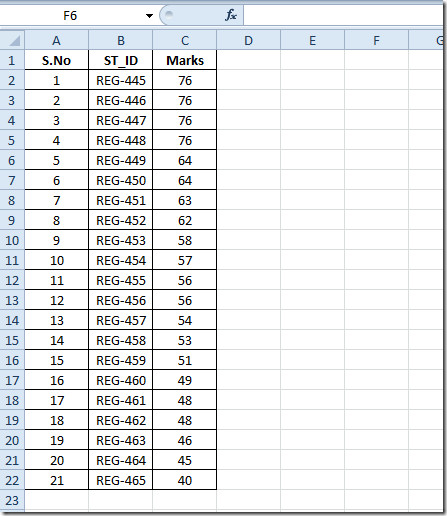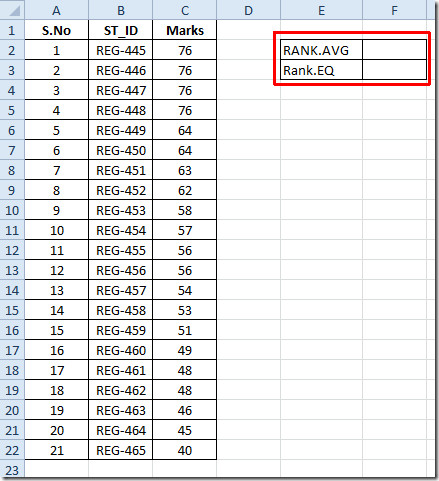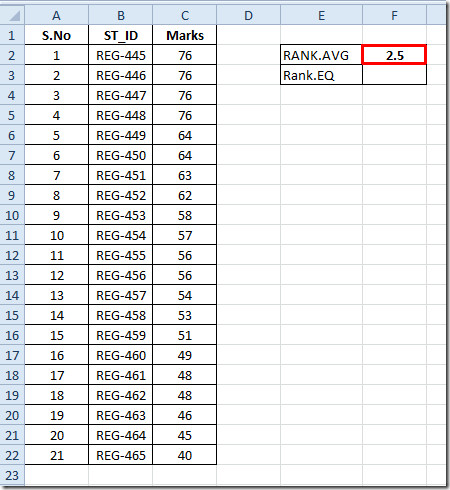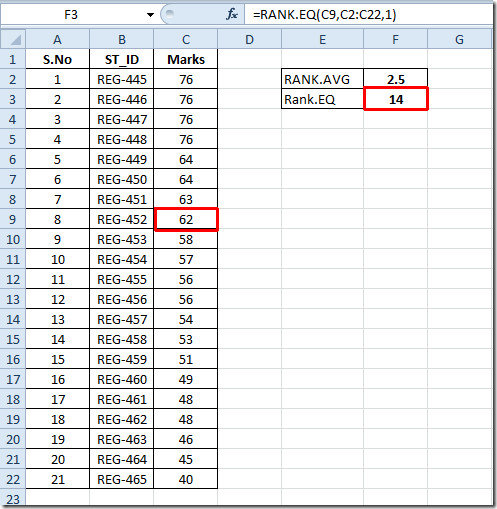# Excel 2010 New RANK functions

Excel 2010 has been augmented with some but powerful features and functions. Even though we have already reviewed the common RANK function, in this post we will be guiding you through two new RANK functions, i.e, RANK.AVG and RANK.EQ introduced in Excel 2010.

Launch Excel 2010 spreadsheet on which you want to check the utility of new ranking functions. For instance we have included spreadsheet containing S.No, ST_ID and Marks.Now we will be using new RANK functions over the dataset for which we will create two new labels adjacent to existing dataset, namely RANK.AVG and RANK.EQ.We will now find out average of ranked values, for this we will use RANK.AVG function. The syntax of RANK.AVG function is same as old RANK function;

=RANK.AVG(number,ref,[order])

In function arguments, number refers to value for which you need to show the rank from the list, the second argument ref refers to the reference list, and [order] refers to two sorting order either ascending or descending.

We will be wiring this function as;

=RANK.AVG(=RANK.AVG(C5,C2:C22,0)

In function argument, C5 is the location of the cell in Marks field (containing 76 value), C2:C22 is the range of the dataset, and 0 is for letting Excel know that we will be sorting in descending order (enter 1 for ascending).

First the function will rank the number then look for any duplicate values in dataset, on finding any duplicate values, it will start taking the average of all duplicated rank values and show the result.Now take a look at RANK.EQ function, Excel wants you to use it instead of going for old-orthodox RANK function.

The syntax is exactly the same as RANK function;

RANK.EQ(number,ref,[order])

We will be writing it as;

=RANK.EQ(C9,C2:C22,1)

In function arguments, C9 is the location of cell in Marks field, C2:C22 refers to range of dataset, and 1 refers to ascending order. It will yield the result, as shown in the screenshot below.You can also check out our previously reviewed Excel functions; WORKDAY.INTL, LARGE, CEILING, TIME, ADDRESS ,FACT (factorial), MAX,MIN, MAXA, MINA and EXACT, Logical Functions, INFO, SUMSQ, DOLLAR, SUMPRODUCT, SUMIF, COUNTIF, VLOOKUP, HLOOKUP ,PMT, & LEN.

1.seo discussion says: